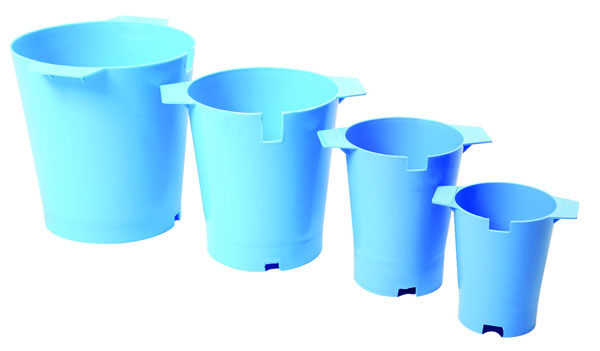Capacity Measure

R65.00 PricePractise Maths Subtraction

R115.00 PriceStudent Clocks – Set Of 12

R741.75 Price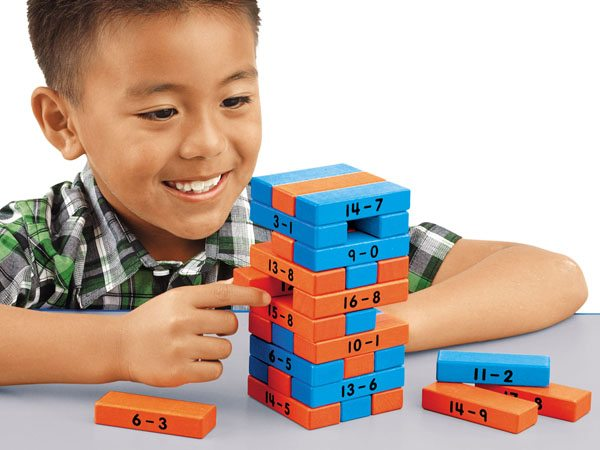Subtraction Tower Of Math Game

R914.25 Price
Sale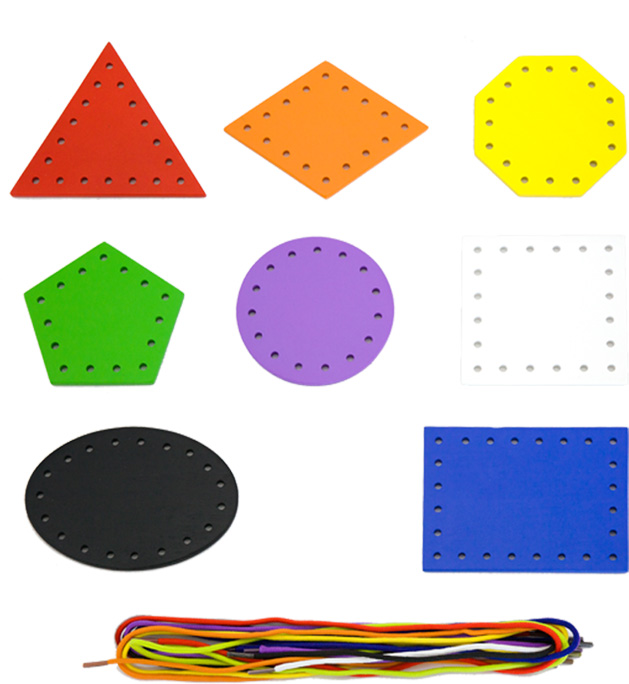Lace And Trace Shapes

R350.00 R295.00 Price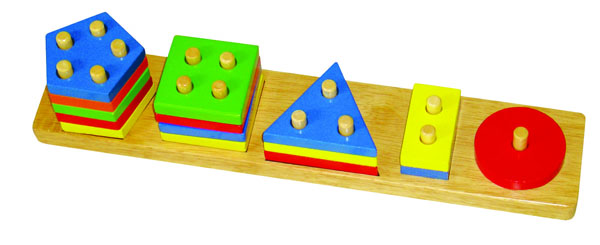Geometric Block Sorter

R350.00 PriceGeometric Stacker

R450.00 PriceR150.00 Price

Learning Maths Beginner Abacus

R300.00 PriceR800.00 Price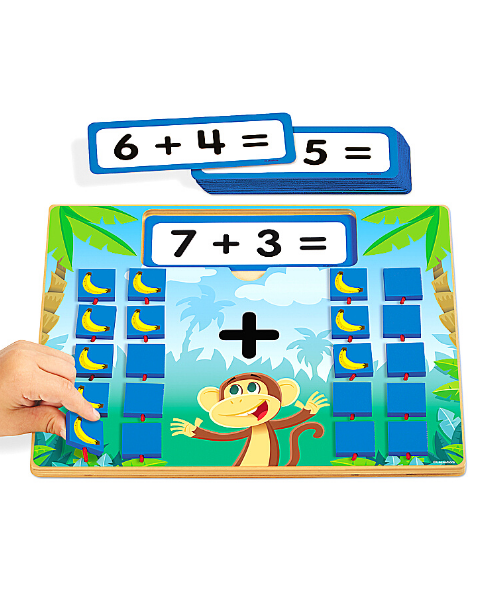Flip And Solve Maths Boards – Addition

R850.00 PriceSquare Sorting Board

R275.00 Price

Showing 1 to 12 of 127 (11 Pages)